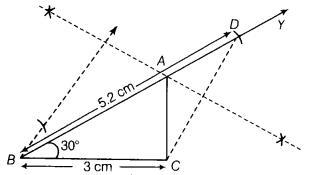# Construct a ∆ABC, in which base BC = 3 cm, ∠B = 30° and AB + AC = 5.2 cm. justify it

Construct a ∆ABC, in which base BC = 3 cm, ∠B = 30° and AB + AC = 5.2 cm. Also justify it.

Given, in ∆ABC, base BC = 3 cm, ∠B = 30° and AB+ AC = 5.2 cm
Steps of construction
(i) First, draw base BC = 3 cm.
(ii) Construct∠YBC = 30° at the point B.
(iii) From ray BY, cut-off line segment BD = AB+ AC = 5.2 cm.
(iv) Join CD.
(v) Draw the perpendicular bisector of CD, which meets BY at A(vi) Join AC. Thus, we get the required ∆ABC.
Justification Since, A lies on the perpendicular bisector of CD.